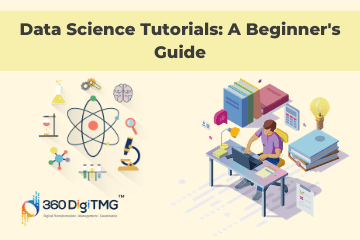Home / Blog / Data Science / Decision Trees and Its Algorithms

# Decision Trees and Its Algorithms

• January 16, 2021
• 2759
• 24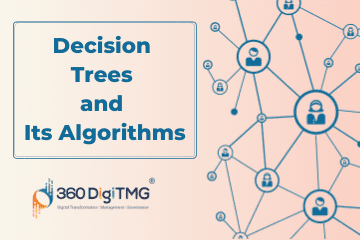### Meet the Author : Mr. Bharani Kumar

Bharani Kumar Depuru is a well known IT personality from Hyderabad. He is the Founder and Director of Innodatatics Pvt Ltd and 360DigiTMG. Bharani Kumar is an IIT and ISB alumni with more than 17 years of experience, he held prominent positions in the IT elites like HSBC, ITC Infotech, Infosys, and Deloitte. He is a prevalent IT consultant specializing in Industrial Revolution 4.0 implementation, Data Analytics practice setup, Artificial Intelligence, Big Data Analytics, Industrial IoT, Business Intelligence and Business Management. Bharani Kumar is also the chief trainer at 360DigiTMG with more than Ten years of experience and has been making the IT transition journey easy for his students. 360DigiTMG is at the forefront of delivering quality education, thereby bridging the gap between academia and industry.

## Introduction

Each internal node of decision trees, which resemble trees, represents a test on a feature or characteristic. As a result of the test on the feature or attribute, each branch that emerges from the root is a branch node. In a Top-Down technique known as a decision tree, the data is divided into additional nodes based on the results of the test on the qualities or features.

### Learn the core concepts of Data Analytics Course video on Youtube:

Numerous machine learning algorithms, including Boosting, Bagging, and Random Forest, are said to be based on Decision Tree. Leo Breiman, a statistician at the University of California, made the initial presentation of it.

A supervised, non-parametric machine learning technique called a decision tree is utilised for both classification and regression. Due to the fact that it divides the characteristics into smaller and smaller subgroups, it is often known as Divide and Conquer. Only axis-parallel splits are possible.

### Decision Trees are represented as Nodes:

• Root Node
• Branch / Internal Node
• Leaf / Terminal Node
• A root node is represented as a Rectangle or a Square. ( or )
• A branch node is represented as a Circle
• Leaf node is represented as a Triangle or a dot. ( or )

• Types of Decision Trees
• Decision Tree-based Ensemble techniques like:
• Bagging
• Random Forest
• Boosting
• Hyper Parameters
• Conclusion

### Types of Decision Trees:

There are two types of Decision Trees that are based on the output variable, namely; Categorical and Continuous.

## Categorical Variable Decision Tree:

This particular decision tree uses a categorical output variable, such as "Yes" or "No," "True" or "False," "Attrite" or "Not Attrite," etc. We have output variables as a factor value with fewer alternatives in categorical decision trees.

Example: Classifying the salaries of employees in a company as ‘High’, ‘Medium’ and ‘Low’. The tree will learn from these features and further split the tree into lower levels.

## Continuous Variable Decision Tree:

This particular sort of decision tree has a continuous output variable. Additionally, the anticipated values will be continuous.

Example: Salaries of employees, Sales information of a store, etc.

Each branch of the decision tree represents a categorization rule. In addition, the Root node determines which classification rules are created and which rules may alter greatly depending on the Root node. Therefore, picking the right Root node is crucial.

Decision trees come with built-in interpretation. The Shallow Machine Learning Model is another name for it. The decision tree uses C 5.0, the most recent version, which offers commercially available parallel computing features.

Variables with high Information Gain should be chosen as Root nodes. We can also use Gini Index and Chi-Square instead of Information Gain for deciding the root and branch nodes. The feature that measures the quality of data split in a Decision tree is with the Gini Impurity value and the entropy of the information gain.

Iterative Dichotomiser3, C4.5 is a successor of ID3 which was widely used earlier and present C5.0 are some of the versions of the decision tree algorithms. Decision Trees are also called CART (Classification and Regression Trees)

It's crucial to explain Greedy Algorithms while talking about the decision tree. In this process, a tree is built from the top down. The variables are categorical, and the method will discretize the data into buckets if it is continuous. Recursively, the input data is divided up based on chosen qualities. Each node's properties are chosen for the test data based on heuristic or statistical criteria.

Entropy or Gini Index values determine the Decision Tree's stopping criterion for splitting; values between 0 and 1 indicate an impure split and 0 indicate a pure split, respectively. When an Entropy value is 0 or a pure split, further splitting is not permitted. We may also determine the relevance of a feature based on its impurity; the greater the impurity value, the more significant the feature.

To build the code, run the various models on the dataset, and evaluate the results, for instance, we are utilising a dataset. We are utilising a dataset for diabetes that has 768 observations and 9 variables.

All of the variables in this dataset are of the "int" data type, and the output variable is a factor with the binary options "YES" or "NO." Where "YES" denotes diabetes and "NO" denotes non-diabetes, respectively.

#### A simple example of Decision Tree on Diabetes dataset 1000 observations:

```Diabetes\$Class.variable = as.factor(Diabetes\$Class.variable) table(Diabetes\$Class.variable) #Shuffle data diabetes_rand<-Diabetes[order(runif(768)), ] str(diabetes_rand) #Split the data diabet_train<-diabetes_rand[2:690, ] diabet_test<-diabetes_rand[691:768, ] table(diabetes_rand\$Class.variable) prop.table(table(diabetes_rand\$Class.variable)) prop.table(table(diabet_train\$Class.variable)) prop.table(table(diabet_test\$Class.variable)) install.packages("C50") library(C50) diabetes_model <- C5.0(diabet_train\$Class.variable ~ ., data= diabet_train) diabetes_model<-C5.0(diabet_train[, -9], diabet_train\$Class.variable) ```

```windows() plot(diabetes_model) ```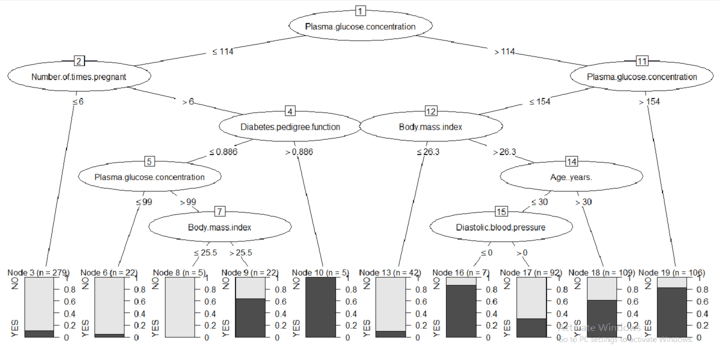```test_res<-predict(diabetes_model, diabet_test) test_acc<-mean(diabet_test\$Class.variable == test_res) test_acc``` ``` >test_acc  0.7435897```

```train_res<-predict(diabetes_model, diabet_train) train_acc<-mean(diabet_train\$Class.variable == train_res) train_acc```

```>train_acc  0.808418```

```table(diabet_train\$Class.variable, train_res) train_acc table(diabet_test\$Class.variable, test_res) test_acc```

```> table(diabet_train\$Class.variable, train_res) train_res NO YES NO 378 70 YES 62 179 > train_acc  0.8```
```> table(diabet_test\$Class.variable, test_res) test_res NO YES NO 39 13 YES 7 19 > test_acc  0.74```

Pruning is the regularisation parameter that will assist in preventing overfitting in the Tree method. Pre- and post-pruning are available.

Pre-pruning allows us to specify up front that the tree should not split past a specific level before the training set is categorised, which has the benefit of halting the tree's growth early. It stays away from the tree splits' intricacy.

Post pruning, also known as backward pruning, is the most often utilised technique. In this pruning, the model first permits the tree to reach its full potential before applying pruning by cutting away any extra branches.

### Example with Pruning with movies dataset:

```diabetes=read.csv(file.choose()) str(diabetes) diabetes\$Class.variable=as.factor(diabetes\$Class.variable) library(caTools) set.seed(9) split=sample.split(diabetes\$Class.variable, SplitRatio = 0.8) diabetes_train=subset(diabetes, split == TRUE) diabetes_test=subset(diabetes, split == FALSE) library(rpart) library(C50) model=rpart(diabetes_train\$Class.variable ~ ., data=diabetes_train, control = rpart.control(cp=0, maxdepth = 3) ) diabetes_model=C5.0(diabetes_train\$Class.variable ~ ., data = diabetes_train, maxdepth = 3) library(rpart.plot) rpart.plot(model, box.palette = "auto", digits = -3)```

### Applying Pre Pruning on the tree with depth=3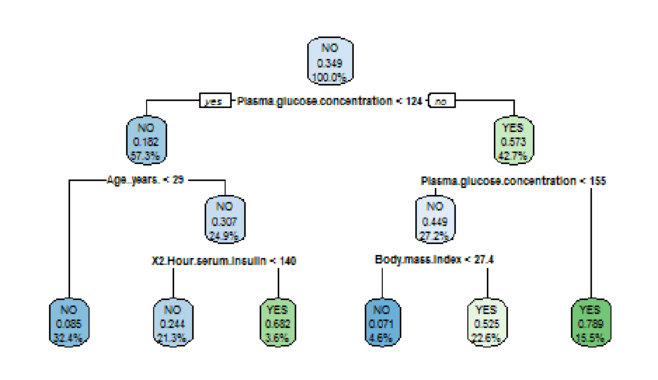### Full Tree with C5.0 model

`plot(diabetes_model)`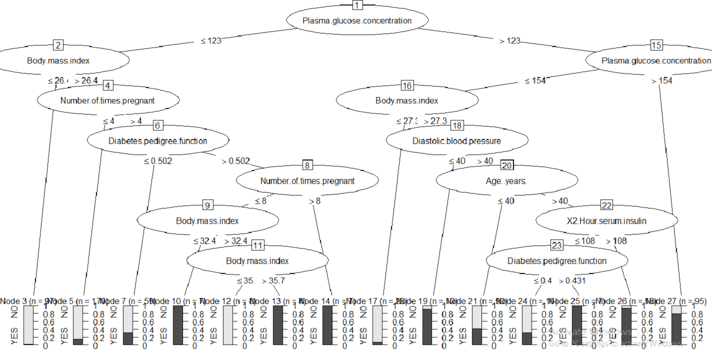### Plotting without Pruning with rpart:

```# Grow the tree fully with rpart fullmodel=rpart(diabetes_train\$Class.variable ~ ., data=diabetes_train, control = rpart.control(cp=0) ) rpart.plot(fullmodel, box.palette = 'auto', digits = -3)```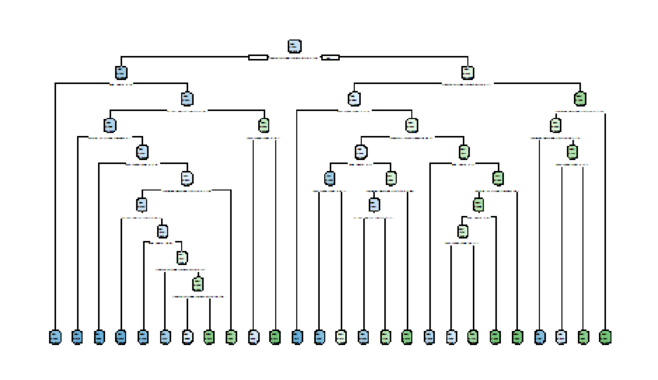```plotcp(fullmodel) plotcp(model)```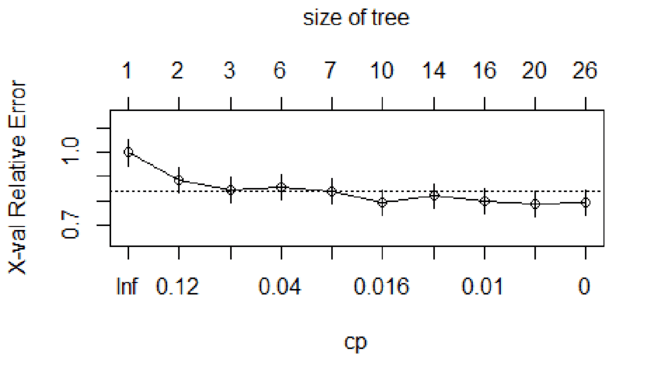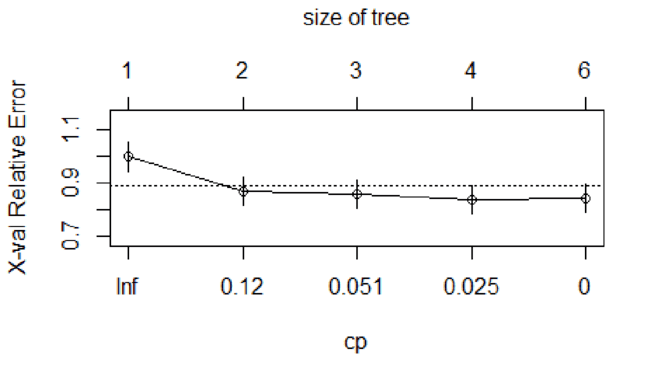### Applying Post Pruning on the rpart applied tree with min CP :

```#pruning the tree with the min CP value mincp=fullmodel\$cptable[which.min(fullmodel\$cptable[, "xerror"]), "CP"] model_prune_1=prune(fullmodel, cp=mincp) rpart.plot(model_prune_1, box.palette = 'auto', digits = -3)```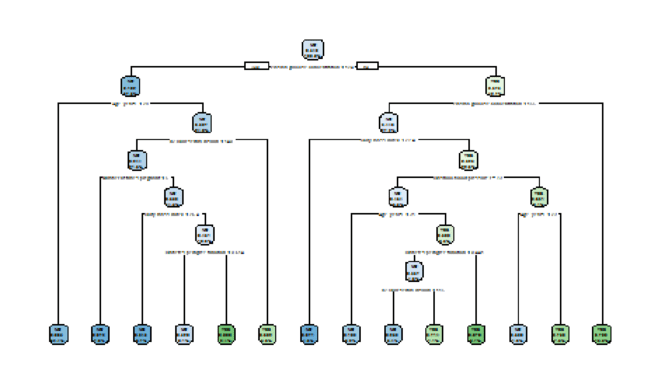### Applying post Pruning with our CP value:

```#using our own CP value for pruning model_prune_2=prune(fullmodel, cp=0.02) rpart.plot(model_prune_2, box.palette = 'auto', digits = -3)```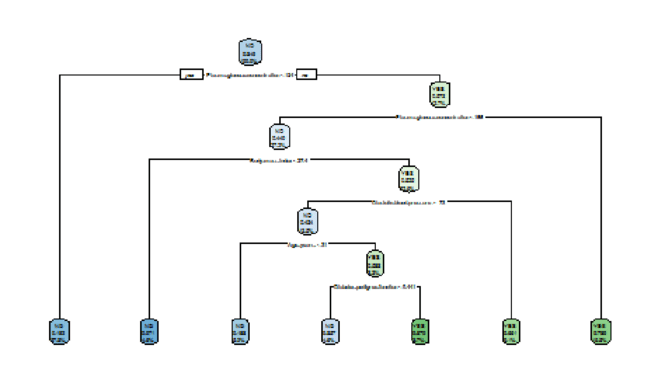Continuous data may be subjected to regression, while categorical data cannot be subjected to regression. The output variable in the diabetes dataset we used as an example is categorical, hence we are unable to determine the RMSE values and the accompanying accuracy for the pruning example.

### Hyperparameters in Decision Tree

Hyperparameter Input Values Default Value
max_depth Integer or None, Optional None
min_samples_split Integer, Float, Optional 2
min_samples_leaf Integer, Float, Optional 1
min_weight_fraction_leaf Float, Optional 0
max_features Int, Float, string or None, Option None
random_state Int, RSI or None, Optional None
min_impurity_decrease Float, Optional 0

By default, the decision tree method is a boosting algorithm. However, decision trees may also be used independently, or as a non-ensemble approach.

## The Disadvantage of Decision Tree

When we have a feature with too many levels it will not work that well, it will get biased with those features. When we have a variable with too many levels the model will learn those features only leaving the other features. Decision trees are ‘greedy’ and identify the solutions locally and they cannot be the solution globally.

## Ensemble Methods

Multiple models are trained using the ensemble machine learning process to provide superior outcomes. When the weak models are appropriately merged, the ensemble method's key benefit is that we can obtain a model that is more accurate.

Multiple machine learning models are aggregated using ensemble learning models, improving performance overall. The reasoning behind this is that each model utilised is poor when used alone, but strong when used as part of an ensemble. With Random Forests, a lot of Decision Trees are employed as the weak elements and their outputs are aggregated to produce the strong ensemble.

The fundamental idea behind ensemble approaches is that by combining several models and methodologies into one model, we may get superior results. We have ensemble ways to solve the conundrum and choose the best machine learning model to use. These methods may be applied to time series, survival, classification, regression, and diagnosis.

By using Ensemble techniques, we can overcome biases and Variances which is the resultant of overfitting, these techniques work well with large and non-linear data sets.

In Ensemble Models we have Boosting, Bagging and Random Forest. The Ensemble models perform better than Decision tree as they consume less time in building the model and weak learners are aggregated.

### Bagging:

Bagging is Bootstrap Aggregating, and the method is part of a Machine Learning Ensemble. It is used with tree-based algorithms, while decision tree models are used with tree-based models. This helps us increase accuracy and stability. This also applies to algorithms that aren't tree-based.

Since its introduction in 1994, this model has mostly been utilised to reduce output volatility and prevent overfitting. Bagging aids in bringing down the variances. Both classification and regression may be done using this model.

For classification models, we either accept the most popular class, known as "hard voting," or all possible classes are considered, with the highest average selected as the output, known as "soft voting." This is referred to as bootstrap aggregation.

We repeatedly employ the same algorithm in Bagging. Every time the data is divided, a decision tree is used. Bagging is a homogeneous ensemble strategy that takes weak learners into account individually and in tandem to produce a model with less variation. Because bootstrap models are often independent and distributed equally, the output of the typical weak learner won't change; instead, the variance will be reduced.

Bagging is a completely data-specific algorithm. As informed above bagging technique reduces overfitting and increases accuracy. Further, the missing values in the dataset will not affect the algorithm performance.

One of the drawbacks of bagging is that the final prediction is dependent on the mean predictions from the subset trees.

### Example of Bagging using Diabetes dataset:

```x_train, x_test, y_train, y_test = train_test_split(predictors, target, test_size = 0.2, random_state=0) from sklearn import tree clftree = tree.DecisionTreeClassifier() from sklearn.ensemble import BaggingClassifier bag_clf = BaggingClassifier(base_estimator = clftree, n_estimators = 500, bootstrap = True, n_jobs = 1, random_state = 53) bag_clf.fit(x_train, y_train) from sklearn.metrics import accuracy_score, confusion_matrix confusion_matrix(y_test, bag_clf.predict(x_test)) accuracy_score(y_test, bag_clf.predict(x_test)) confusion_matrix(y_train, bag_clf.predict(x_train)) accuracy_score(y_train, bag_clf.predict(x_train))```

### Train and Test Accuracy:

```In : confusion_matrix(y_test, bag_clf.predict(x_test)) Out: array([[92, 15], [13, 34]], dtype=int64) In: accuracy_score(y_test, bag_clf.predict(x_text)) Out:0.8181818181818182 In:confusion_matrix(y_train,bag_clf.predict(x_train)) Out: array([[393, 0], [0, 221]], dtype=int64) In:accuracy_score(y_train, bag_clf.predict(x_train)) Out:1.0```

### Hyperparameters in Bagging:

Hyperparameter Input Values Default Value
base_estimator Int Decision tree
n_estimators Int 10
random_state seed None
n_jobs Int, None None

### Random Forest:

It is an ensemble-based supervised learning approach that applies to both regression and classification models. In 1995, Tin Kam Ho from Bell Laboratories first presented it.

A forest is a collection of strong learners that includes several trees. In this, we often use Bootstrap sampling (which refers to sampling with replacement). An improvement on bagging is random forest. We create tree-based models for each sample, mostly using decision trees. Because we base each tree on a different sample of data, each tree that makes up Random Forest is unique. Additionally, Random Forest minimises overfit, making it more accurate than a single decision tree. We will subsample the columns or inputs in addition to the observations to lessen the correlation between the trees. Decision trees are grouped together in Random Forest, which also selects the columns at random. With this kind of feature-based sampling, the decision-making process is strengthened, the similarity between the trees is significantly decreased, and the output variances are increased.

The primary distinction between Bagging and Random Forest is that, in Bagging, the observations are randomly divided into train and test data; with a Random Forest, however, not only are the observations randomly divided, but also the variables for the sample.

It is a versatile algorithm that can solve overfitting problems. It can handle high dimensional and missing data well. As it takes a maximum number of trees and makes it difficult to interpret and makes the computation expensive and time-consuming.

### Example of Random Forest with the Diabetes Dataset:

```from sklearn.preprocessing import scale diabetes_op = diabetes[" Class variable"] diabetes_num = diabetes.drop(" Class variable", axis = 1) diabetes_std = pd.DataFrame(scale(diabetes_num)) diabetes_final = pd.concat([diabetes_std, diabetes_op], axis=1) predictors = diabetes_final.loc[:, diabetes_final.columns!=" Class variable"] target = diabetes_final[" Class variable"] # Class Variable ('Y' OUTPUT ) # Dta Split from sklearn.model_selection import train_test_split x_train,x_test,y_train,y_test=train_test_split(predictors, target, test_size = 0.2, random_state=0) # Train Test partition of the data from sklearn.model_selection import train_test_split x_train, x_test, y_train, y_test = train_test_split(predictors, target, test_size = 0.2, random_state=0)```

### Evaluating Test Accuracy:

```from sklearn.ensemble import RandomForestClassifier rf_clf = RandomForestClassifier(n_estimators=500, n_jobs=1, random_state=42) rf_clf.fit(x_train, y_train) from sklearn.metrics import accuracy_score, confusion_matrix confusion_matrix(y_test, rf_clf.predict(x_test)) accuracy_score(y_test, rf_clf.predict(x_test))```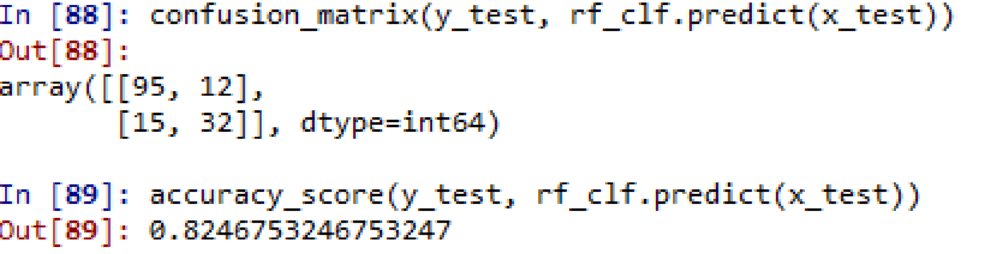### Evaluation using Grid Search:

```from sklearn.model_selection import GridSearchCV rf_clf_grid = RandomForestClassifier(n_estimators=500, n_jobs=1, random_state=42) param_grid = {"max_features": [4, 5, 6, 7, 8, 9, 10], "min_samples_split": [2, 3, 10]} grid_search = GridSearchCV(rf_clf_grid, param_grid, n_jobs = -1, cv = 5, scoring = 'accuracy') grid_search.fit(x_train, y_train) grid_search.best_params_ cv_rf_clf_grid = grid_search.best_estimator_ from sklearn.metrics import accuracy_score, confusion_matrix confusion_matrix(y_test, cv_rf_clf_grid.predict(x_test)) accuracy_score(y_test, cv_rf_clf_grid.predict(x_test))```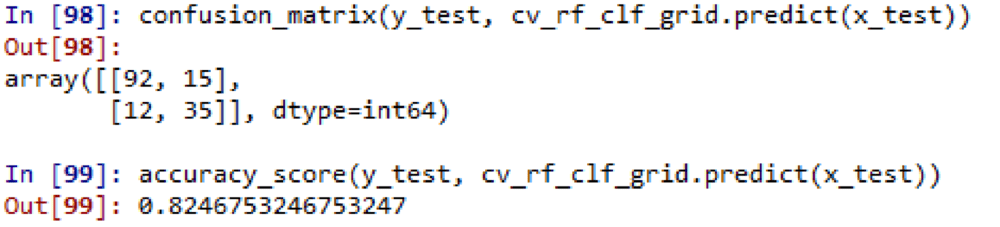### Hyperparameters in Random Forest:

Hyperparameter Input Values Default Value
n_estimators Integer 100
criterion Integer, float Gini
max_depth Integer, None None
max_features Integer, float Auto, sqrt (# of features)
min_samples_leaf Integer 1
n_jobs Integer 1
oob_score Boolean False

### OOB:

OOB: This form of random forest cross-validation uses one-third of the data for validation rather than training, and these kinds of samples are known as OOB (Out of Bag) samples or scores.

### Boosting:

It is one of the ensemble techniques which is used to improve accuracy and performance. In this, it will take a bunch of weak learners or Base learners and combine those weak learners to make a strong learner. In this, the predicting model will learn from the outcomes of the previous predictors.

### There are 2 characteristics in Boosting which are:

• First, we need to run multiple iterations.
• Each iteration focuses on the instances that were wrongly classified by the previous iterations.

The output of the learning algorithms (weak learners) is merged into a weighted sum that reflects the final output, and boosting may be used in conjunction with many other learning algorithms to increase performance.

### Bagging and Boosting difference:

If a single model is overfitted, boosting will not work for overfit models. If the models are overfitting, Bagging is the option. Boosting is good for models which are under fitted or biased.

### We have got 3 Boosting Algorithms:

Adaboost is adaptive in that it modifies succeeding weak learners in favour of individuals who were incorrectly categorised by the prior classifier. There is a trade-off between learning rate and N-estimator in Adaboost; by default, n_estimators are set to 50.

The class of an item might not be accurately predicted by a poor classifier. However, by combining those ineffective classifiers and learning from one another's mistakes, we may create a powerful single model.

Adaboost uses decision stumps in his work. They are nothing more than the trees in a decision tree created using a random forest. The tree, however, is not fully developed in the decision stump; it only has one node and two leaves.

Decision stump refers to a single-split tree is a poor classifier constructed using weighted samples and training data. Each sample's weights are properly categorised in this. We will first assign identical weights to all of the samples for the first decision stump. Make a decision stump for each variable after that, and check to see if it assigns the inputs to the correct classes.

In order to appropriately classify the previously misclassified samples in the upcoming decision stump, we will give them higher weight.

Despite the high weights assigned, the training dataset would probably be more affected by the training dataset. Furthermore, low weight assignments have less of an impact on the training dataset. Individual weights will always fall between 0 and 1, thus the total of all weights will be 1.

It can be used to increase the accuracy of the weak classifier making the results more accurate and reliable. This can be used effectively with less hyper parameter tweaking. Adaboost is very sensitive to noisy data and outliers, this is an advantage in Adaboost.

### Example of AdaBoost using the same dataset:

```from sklearn.ensemble import AdaBoostClassifier ada_clf = AdaBoostClassifier(learning_rate = 0.02, n_estimators = 5000) ada_clf.fit(x_train, y_train) from sklearn.metrics import accuracy_score, confusion_matrix```

### Evaluation on test data:

```#Accuracy on Test Data confusion_matrix(y_test, ada_clf.predict(x_test)) accuracy_score(y_test, ada_clf.predict(x_test))```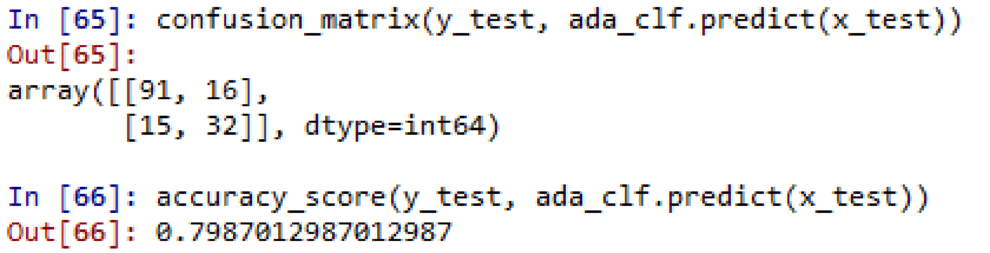### Evaluation on train data:

```#Accuracy on Train Data confusion_matrix(y_train, ada_clf.predict(x_train)) accuracy_score(y_train, ada_clf.predict(x_train))```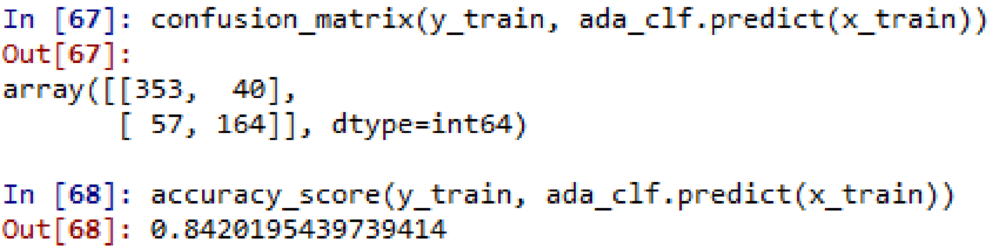Hyperparameter Input Values Default Value
Max_depth Integer 1
base_estimator Object None
n_estimators Integer 50
learning_rate Integer 1
random_state Integer, RS instance, or None None

Jerome H. Friedman invented gradient boosting. Other names for a gradient include slope, rate of change, and derivative. Gradient boosting builds a strong model repeatedly from each of the preceding weak learners. In gradient boosting, the loss is the main focus. Here, the weights are constantly shifting to reduce loss.

There are three sequential steps in Gradient boosting:

• Fit the model to the data
• Fit the model to errors or residuals
• Create a new model

### Gradient boosting algorithm descent steps explained:

Gradient boosting believes that the best possible next model which when combined with the previous Gradient boosting contends that by combining the best subsequent model with the prior one, the overall prediction error may be reduced. Setting goal outcomes for the following model is the fundamental concept in order to reduce mistakes. The output goal for each example in the data is based on how much the feature's forecast has changed, which affects the total prediction inaccuracy.

The next target outcome of the case is a high value if a little adjustment in the forecast for a given case results in a significant decrease in inaccuracy. The error will be reduced by predictions from the new model that are near to their goals. The next intended result of the case is zero if a modest modification in the forecast for a case has no impact on the error. This forecast cannot be altered to reduce the inaccuracy.

It works well with categorical and count data and also handles the missing data well. Sometimes gradient boosting can cause overfitting and increase the outliers.

```x_train, x_test, y_train, y_test = train_test_split(predictors, target, test_size = 0.2, random_state=0) from sklearn.ensemble import GradientBoostingClassifier boost_clf = GradientBoostingClassifier() boost_clf.fit(x_train, y_train) from sklearn.metrics import accuracy_score, confusion_matrix confusion_matrix(y_test, boost_clf.predict(x_test)) accuracy_score(y_test, boost_clf.predict(x_test)) # Various Hyperparameters Tuning boost_clf2 = GradientBoostingClassifier(learning_rate = 0.02, n_estimators = 1000, max_depth = 1) boost_clf2.fit(x_train, y_train) confusion_matrix(y_train, boost_clf2.predict(x_train)) accuracy_score(y_train, boost_clf2.predict(x_train))```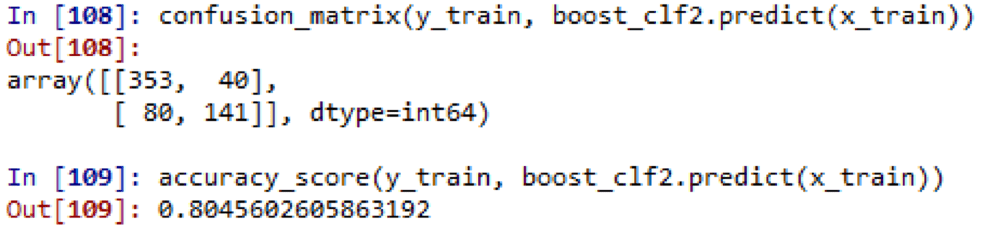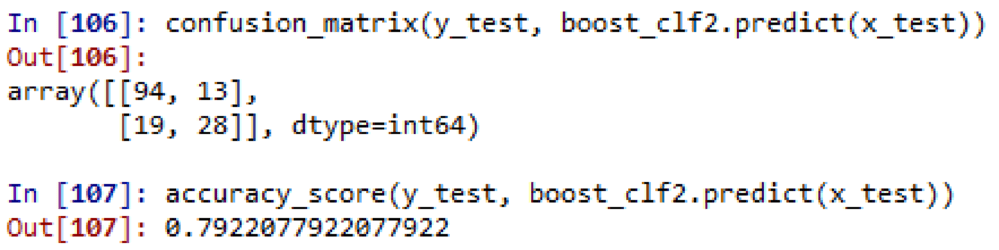Hyperparameter Input Values Default Value
n_estimators Integer 100
max_depth Integer 3
min_samples_split Integer 2
min_samples_leaf Integer 1
Learning_rate Integer 0.1
Subsample Integer, float 1.0
Criterion Integer, float Gini

Instances which were giving error were given more weights and were resampled again It builds each base learner on the previous model loss or error
It uses Decision Stump Gradient boosting uses a Decision Tree of varying depths

### XGB:

The gradient boosting decision tree method is one of the Tree-based Ensemble Techniques that is implemented by XG Boost. Gradient boosting has been improved, and now Classifiers and Regressors may both benefit from XG Boost. It performs well in parallel computing and contains built-in regularisation. It can deal with missing values and provide correct results quickly. Regularisation is one of the learning objectives of XG Boost.

The Execution speed and Model performance of XG boosting are its two primary benefits.

Since it makes use of the GPU, XG Boosting is quicker than other gradient implementations.

Because it has been the favourite algorithm for the winners of multiple Kaggle tournaments, XGBoost has gained a lot of notoriety.

The key features of the XG boost, which keeps it ahead of other algorithms:

• It parallelly works on multiple trees by using all the CPU resources during training.
• Distributed Computing for training very large models using a cluster of machines.
• Out-of-Core Computing for very large datasets that don’t fit into memory.
• Cache Optimization of data structures and algorithm to make the best use of hardware.

### Things that make this algorithm extremely fast:

• Approximate split finding algorithm, this uses quantiles.
• Sparsity aware split finding.
• Parallel computing: XG Boost sorts and compresses the data into blocks which enables parallel computing and expedites the whole process.
• Cache aware access.
• Block compression and sharding.

### Example of XGB with the same Diabetes dataset:

```x_train, x_test, y_train, y_test = train_test_split(predictors, target, test_size = 0.2, random_state=0) import xgboost as xgb xgb_clf = xgb.XGBClassifier(max_depths = 5, n_estimators = 5000, learning_rate = 0.5, n_jobs = -1) xgb_clf.fit(x_train, y_train) from sklearn.metrics import accuracy_score, confusion_matrix confusion_matrix(y_test, xgb_clf.predict(x_test)) accuracy_score(y_test, xgb_clf.predict(x_test)) xgb.plot_importance(xgb_clf) ```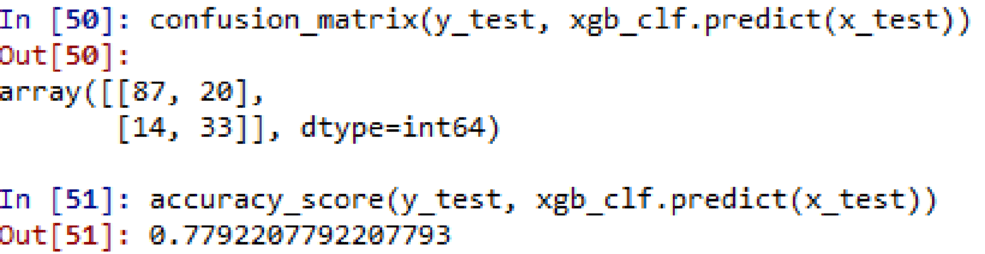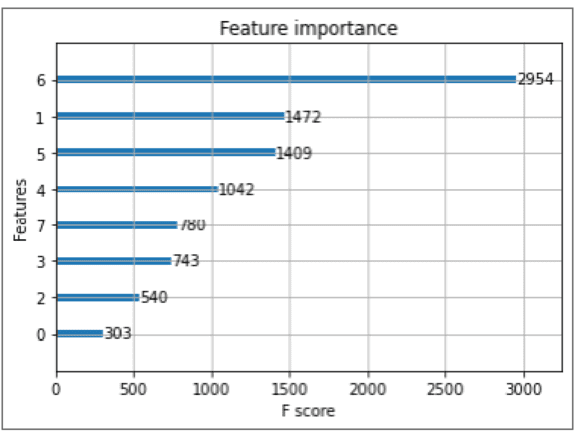```Hyper Parameters for Grid Search xgb_clf = xgb.XGBClassifier(n_estimators = 500, learning_rate = 0.1, random_state = 42) param_test1 = {'max_depth': range(3,10,2), 'gamma': [0.1, 0.2, 0.3], 'subsample': [0.8, 0.9], 'colsample_bytree': [0.8, 0,9], 'rag_alpha': [1e-2, 0.1, 1]} # Using Grid-Search from sklearn.model_selection import GridSearchCV grid_search = GridSearchCV(xgb_clf, param_test1, n_jobs = -1, cv = 5, scoring = 'accuracy') grid_search.fit(x_train, y_train) cv_xg_clf = grid_search.best_estimator_ # Using hyperparameter for Testing accuracy_score(y_test, cv_xg_clf.predict(x_test)) grid_search.best_params_```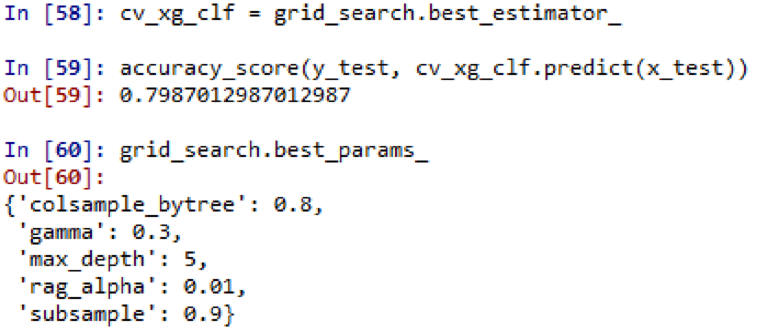The accuracy has gone up from 77 to 78 percent because to Grid search.

Run the following command as "pip install XGBoost" on the Anaconda CMD prompt if you are unable to load the XG Boost in Python. You might also run your code in Google Collab as an alternative.

### Hyperparameters in XG Boost:

Hyperparameter Input Values Default Value
n_estimators Integer 100
max_depth Integer 6
Subsample Integer, float 1
Eta Integer, float 0.3
min_child_weight Integer 1
gamma Integer, Float 0
alpha Integer, float 0
max_delta_step Integer, float 0
scale_pos_weight Integer, float 1
Lambda Integer, float 1
colsample_byleve Integer, float 1
colsample_bytree Integer, float 1

Grid Search Techniques are used when there are several hyperparameters, such as the ones mentioned above. In this situation, the data is divided into Training, Validation, and Test.

Comparing all the above models depends on the business problem and various other parameters like input variables and the outputs to determine the best model that suits your requirement. However, XGB can outdo the other models in most of the scenarios when it comes to performance coupled with time. Also one can decide on the best model based on one’s experience and experimentation.

### Conclusion:

In order to acquire the greatest results from the data sets we are working with, we need to experiment with all of these methods, work on them extensively, and try to utilise alternative values.

Only when you experiment with changing the hyperparameter settings to find the optimal model that is in line with the business aim and the restrictions that we have inferred from the business challenge supplied by the customer will you obtain the best results from the aforementioned models.

Click here to learn Data Science Course, Data Science Course in Hyderabad, Data Science Course in Bangalore

### Data Analyst Courses in Other Locations